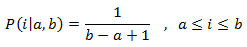class template
<random>

# std::uniform_int_distribution

`template <class IntType = int> class uniform_int_distribution;`
Uniform discrete distribution
Random number distribution that produces integer values according to a uniform discrete distribution, which is described by the following probability mass function:This distribution produces random integers in a range [a,b] where each possible value has an equal likelihood of being produced. This is the distribution function that appears on many trivial random processes (like the result of rolling a die).

The distribution parameters, a and b, are set on construction.

To produce a random value following this distribution, call its member function operator().

For a discrete distribution that can have different probabilities for each possible value, see discrete_distribution.

### Template parameters

IntType
An integer type. Aliased as member type result_type.
By default, this is int.

### Member types

The following aliases are member types of uniform_int_distribution:

member typedefinitionnotes
result_typeThe first template parameter (IntType)The type of the numbers generated (defaults to int)
param_typenot specifiedThe type returned by member param.

### Example

 ``12345678910111213141516171819202122232425`` ``````// uniform_int_distribution #include #include int main() { const int nrolls = 10000; // number of experiments const int nstars = 95; // maximum number of stars to distribute std::default_random_engine generator; std::uniform_int_distribution distribution(0,9); int p={}; for (int i=0; i

Possible output:
 ```uniform_int_distribution (0,9): 0: ********* 1: ********* 2: ********* 3: ********* 4: ********* 5: ********* 6: ********* 7: ********* 8: ********* 9: ********* ```